• 1.坐标系、坐标轴、刻度绘制 代码： # -*- coding: utf-8 -*- """ Created on Wed May 15 16:09:38 2019 @author: mzl """ import matplotlib as mpl import matplotlib.pyplot as plt # 下面代码打印出坐标系、...
1.坐标系、坐标轴、刻度绘制

代码：
# -*- coding: utf-8 -*-
"""
Created on Wed May 15 16:09:38 2019
@author: mzl
"""
import matplotlib as mpl
import matplotlib.pyplot as plt

# 下面代码打印出坐标系、坐标轴和刻度。
fig = plt.figure()
ax = fig.add_subplot(1, 1, 1)
plt.show()

xax = ax.xaxis
yax = ax.yaxis

print('fig.axes:', fig.axes, '\n')
print('ax.xaxis:', xax )
print('ax.yaxis:', yax, '\n' )
print('ax.xaxis.majorTicks:', xax.majorTicks, '\n')
print('ax.yaxis.majorTicks:', yax.majorTicks, '\n')
print('ax.xaxis.minorTicks:', xax.minorTicks)
print('ax.yaxis.minorTicks:', yax.minorTicks)

结果显示：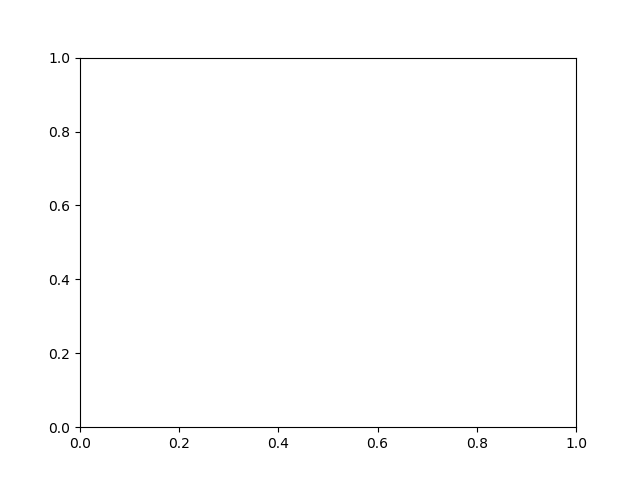展开全文python
• ## matplotlib设置坐标轴

万次阅读 多人点赞 2017-05-04 15:59:30
在使用matplotlib模块时画坐标图时，往往需要对坐标轴设置很多参数，这些参数包括横纵坐标轴范围、坐标轴刻度大小、坐标轴名称等 在matplotlib中包含了很多函数，用来对这些参数进行设置。plt.xlim、plt.ylim 设置...
在使用matplotlib模块时画坐标图时，往往需要对坐标轴设置很多参数，这些参数包括横纵坐标轴范围、坐标轴刻度大小、坐标轴名称等
在matplotlib中包含了很多函数，用来对这些参数进行设置。

plt.xlim、plt.ylim                            设置横纵坐标轴范围
plt.xlabel、plt.ylabel        设置坐标轴名称
plt.xticks、plt.yticks设置坐标轴刻度

以上plt表示matplotlib.pyplot

例子


#导入包
import matplotlib.pyplot as plt
import numpy as np

#创建数据
x = np.linspace(-5, 5, 100)
y1 = np.sin(x)
y2 = np.cos(x)

#创建figure窗口
plt.figure(num=3, figsize=(8, 5))
#画曲线1
plt.plot(x, y1)
#画曲线2
plt.plot(x, y2, color='blue', linewidth=5.0, linestyle='--')
#设置坐标轴范围
plt.xlim((-5, 5))
plt.ylim((-2, 2))
#设置坐标轴名称
plt.xlabel('xxxxxxxxxxx')
plt.ylabel('yyyyyyyyyyy')
#设置坐标轴刻度
my_x_ticks = np.arange(-5, 5, 0.5)
my_y_ticks = np.arange(-2, 2, 0.3)
plt.xticks(my_x_ticks)
plt.yticks(my_y_ticks)

#显示出所有设置
plt.show()

结果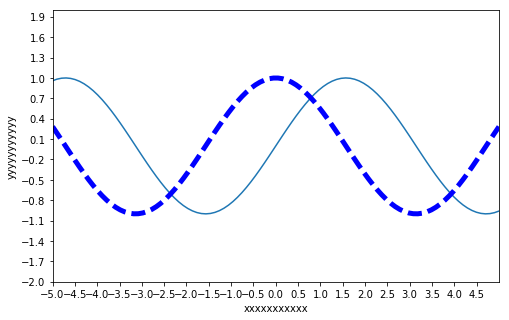展开全文函数
• 早上想把用matplotlib画的x轴的坐标单位标上去，但是找了半天没找到方法，谁有好的方法请告诉我。 找到了调整x轴坐标刻度的方法，也就是修改坐标轴的密度： plt.xticks([1,2,3,4]) 参考：Python matplotlib - ...
早上想把用matplotlib画的x轴的坐标单位标上去，但是找了半天没找到方法，谁有好的方法请告诉我。
找到了调整x轴坐标刻度的方法，也就是修改坐标轴的密度：
plt.xticks([1,2,3,4])

参考：Python matplotlib - setting x-axis scale - Stack Overflow
然后我亲自提了一个问题：python - how to add matplotlib axis unit - Stack Overflow
我现在不追求把坐标轴标上单位，而是在每个数字后面加上单位。
做法如下：
import matplotlib.pyplot as plt
import matplotlib.ticker as mticker
x = [i * 2872155 for i in range(1, 11)]
y = [0.219, 0.402,  0.543,  0.646,0.765,  0.880,1.169, 1.358,1.492,1.611]

plt.gca().yaxis.set_major_formatter(mticker.FormatStrFormatter('%.1f s'))
plt.plot(x, y)

效果图如下：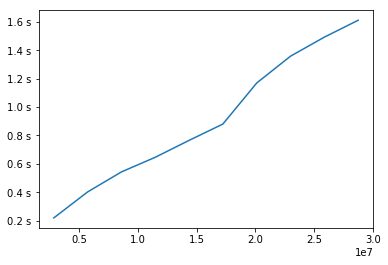展开全文• 如下所示： <span xss=removed>>>> import numpy as np</span> >>> import matplotlib.pyplot as plt >>> x=np.arange(-5,5,0.01) >>> y=x**3 >>> plt.axis([-6,6,-10,10]) [-6, 6, -10, 10] ...出来
• 本来是想让横轴显示1-20的整数，刻度之间间隔1，但是出图来却显示有浮点数 plt.title('train loss') plt.plot(np.arange(1,len(losses)+1), losses) plt.plot(np.arange(1,len(eval_losses)+1), eval_losses) 经...
本来是想让横轴显示1-20的整数，刻度之间间隔1，但是画出图来却显示有浮点数
plt.title('train loss')
plt.plot(np.arange(1,len(losses)+1), losses)
plt.plot(np.arange(1,len(eval_losses)+1), eval_losses)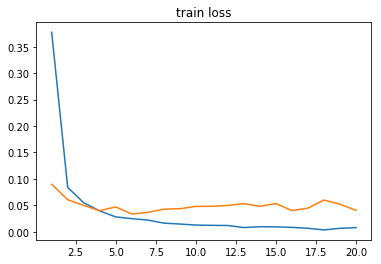经查资料，找到两种方法：
第一种是通过MultipleLocator类来实现
from matplotlib.pyplot import MultipleLocator #导入此类，设置坐标轴间隔
plt.title('train loss')

x_major_locator=MultipleLocator(1)
ax=plt.gca()
ax.xaxis.set_major_locator(x_major_locator)

plt.plot(np.arange(1,len(losses)+1), losses)
plt.plot(np.arange(1,len(eval_losses)+1), eval_losses)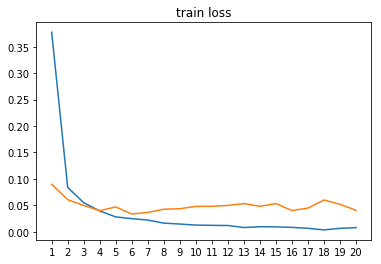第二种是将横轴坐标范围转换成字符串
plt.title('train loss')
plt.plot(np.arange(1,len(losses)+1).astype(np.str), losses)
plt.plot(np.arange(1,len(eval_losses)+1), eval_losses)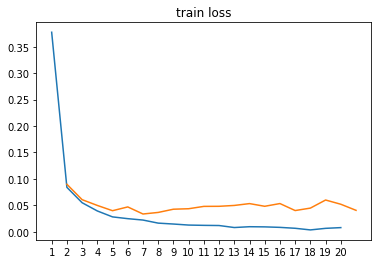展开全文python 可视化
• 在使用matplotlib模块时画坐标图时，往往需要对坐标轴设置很多参数，这些参数包括横纵坐标轴范围、坐标轴刻度大小、坐标轴名称等在matplotlib中包含了很多函数，用来对这些参数进行设置。我们可以对坐标轴进行设置，...
• >>> import numpy as np >>> import matplotlib.pyplot as plt >>> x=np.arange(-5,5,0.01) >>> y=x**3 >>> plt.axis([-6,6,-10,10]) [-6, 6, -10, 10] >>> plt.plot(x,y) ...另外坐标轴还有另一种python 代码
• 容易的事。例如下面的代码： 绘制结果为： ...虽然确实几行代码就出了正弦曲线，但是...虽然这个axisartist能够很方便地自定义坐标轴的位置和完美地设置箭头，但对于更多属性的设置有点乏力，很多参数的微调还...python 可视化
• 车辆路径规划问题的研究一般较常遇到需要出车辆路径示意图，已知有每辆车的真实坐标序列，那么如何利用在一个空白的坐标轴出路径呢？ 1.准备 1.1 matplotlib引入 一般情况下只引入plt就行了，但是我这里因为要...
• 图书推荐：《Python程序设计基础与应用》...===============使用Python+numpy+matplotlib这样的组合，如果要绘制一条正弦曲线，是很容易的事。例如下面的代码：绘制结果为：虽然确实几行代码就出了正弦曲线，但...
• 一、问题描述 具体问题是： 用python库matplotlib进行数据的图表展示； 横坐标是自定义统计值； 保存矢量图（svg），保存后发现横坐的... 二、解决办法 解决思路： 查一下相关坐标轴的参数。 解决办法： 方法1： 通...
• 我在这里用annotate给出了一个画坐标轴的办法。效果如下： 代码实现 import matplotlib.pyplot as plt import numpy as np x_np_list = np.arange(-10, 10, 0.01) y_list =  * len(x_np_list) # 创建元素相同的...python
• 设置坐标轴​ 还记得上次的那条“项链”嘛？结尾的时候有说过，这些是新手村礼包，还有很多其他值得探索的地方呢，那么就一起来康康还有哪些意想不到的操作吧(包括但不限于折线图哦，很多操作再其他图中也是可以...
• 设置坐标轴 ​ 还记得上次的那条“项链”嘛？结尾的时候有说过，这些是新手村礼包，还有很多其他值得探索的地方呢，那么就一起来康康还有哪些意想不到的操作吧(包括但不限于折线图哦，很多操作再其他图中也是可以...可视化 数据可视化 python
• 简介使用matplotlib绘图，一个非常基础的问题就是要搞清楚画布和坐标轴。只有真正了解这两个概念，才能获得对整个绘图过程的控制权。画布(Figure)想要绘图就需要一张画布，所有的内容都是放在画布上面的。通过Figure...
• 图书推荐：《Python程序设计基础与应用》...===============使用Python+numpy+matplotlib这样的组合，如果要绘制一条正弦曲线，是很容易的事。例如下面的代码：绘制结果为：虽然确实几行代码就出了正弦曲线，但...
• 设置坐标轴​ 还记得上次的那条“项链”嘛？结尾的时候有说过，这些是新手村礼包，还有很多其他值得探索的地方呢，那么就一起来康康还有哪些意想不到的操作吧(包括但不限于折线图哦，很多操作再其他图中也是可以...
• 设置坐标轴​ 还记得上次的那条“项链”嘛？结尾的时候有说过，这些是新手村礼包，还有很多其他值得探索的地方呢，那么就一起来康康还有哪些意想不到的操作吧(包括但不限于折线图哦，很多操作再其他图中也是可以...
• plt.xlim(-10,10) #x坐标轴范围-10~10 plt.ylim(-10,10) #第一个参数为标记文本，第二个参数为标记对象的坐标，第三个参数为标记位置 plt.annotate('(2,2)', xy=(2,2), xytext=(2.5,2.5)) plt.annotate('(-2,-2)',
• import matplotlib.pyplot as pltimport numpy as npdef 画图():#plt.grid() #显示网格#plt.rcParams['axes.unicode_minus'] = False #可显示负号plt.title("十字坐标图示")fig=plt.figure(1,dpi=160)ax=plt.gca()#...
• 在使用matplotlib模块时画坐标图时，往往需要对坐标轴设置很多参数，这些参数包括横纵坐标轴范围、坐标轴刻度大小、坐标轴名称等 在matplotlib中包含了很多函数，用来对这些参数进行设置。 我们可以对坐标轴进行...
• 在使用matplotlib模块时画坐标图时，往往需要对坐标轴设置很多参数，这些参数包括横纵坐标轴范围、坐标轴刻度大小、坐标轴名称等在matplotlib中包含了很多函数，用来对这些参数进行设置。我们可以对坐标轴进行设置，......